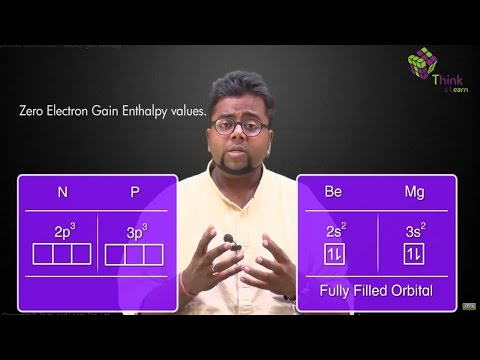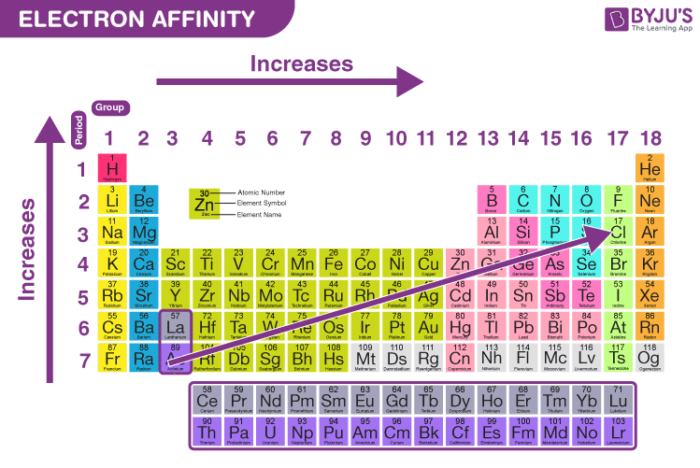# Electron Affinity

## What is Electron Affinity?

Electron affinity is defined as

The amount of energy released when an electron is added to a neutral atom to form an anion.

The electron affinity is the potential energy change of the atom when an electron is added to a neutral gaseous atom to form a negative ion. So the more negative the electron affinity the more favourable the electron addition process is. Not all elements form stable negative ions in which case the electron affinity is zero or even positive.

## Recommended Videos## Periodic Trends

The amount of energy released when an electron is added to a neutral atom to form an anion is called electron affinity. Electron affinities are difficult to measure.

• Electron affinity increases going left to right across a period because of increased nuclear attraction.
• Going down the group the electron affinity should decrease since the electron is being added increasingly further away from the nucleus. Electron becomes less tightly bound and can be easily removed
$$\begin{array}{l}Electron\ affinity = \frac{1}{Atomic\ Size}\end{array}$$

In a period, as we move from left to right the atomic size decreases due to the increase in the nuclear force hence the electron gain enthalpy increases. Whereas while moving down a group in the periodic table, the atomic size increases thereby causing a decrease in the value of electron gain enthalpy.

The first electron affinity is always exothermic that is negative. The second electron affinity of the same element will be positive or endothermic. This is so because the second electron has to be forced to enter the mono negative ion. The electron affinity cannot be determined directly but is obtained indirectly from the Born-Haber cycle.

## Factors Affecting Electron Affinity

The general factors that affect electron affinity are listed below.

• $$\begin{array}{l}Electron\ affinity = \frac{1}{Atomic\ Size}\end{array}$$
• $$\begin{array}{l}Electron\ affinity = Effective\ Nuclear\ Charge\end{array}$$
• $$\begin{array}{l}Electron\ affinity = \frac{1}{Screening\ Effect}\end{array}$$
• $$\begin{array}{l}Reactivity\ of\ non-metals = Electron\ affinity\end{array}$$
• $$\begin{array}{l}Oxidising\ power\ of\ element = Electron\ affinity\end{array}$$

1. Atomic size: If the atomic size is small, then there will be greater electron gain enthalpy because the effective nuclear forces will be greater in the smaller atoms and the electrons will be held firmly.

2. Nuclear charge: The greater the nuclear charge, more will be the value of electron gain enthalpy because  increase in nuclear change increase nuclear force on valence electrons.In general electron affinity follows the following trends:

Halogens > Oxygen family > Carbon family > Nitrogen family > Metals of group 1 and 13 > Metals of group 2

## Electron Affinity of Halogens

Ionization potential is the energy required to remove an electron from a gaseous atom. Energy is supplied for removing an electron implies that energy will be released if an extra electron is added to the atom. The amount of energy released when a neutral atom in its gaseous state accepts an electron and gets converted into a negatively charged ion is known as electron affinity.

$$\begin{array}{l}X (g) ~+~ e^-~ \rightarrow ~X^- ~(g) ~+ ~E.A.\end{array}$$

Here X is any element in the gaseous state and E.A. is the electron affinity. The unit of electron affinity is electron volts per atom or kilojoule per mole. It is represented by a negative sign [-] for example,

$$\begin{array}{l}Cl ~(g) ~+ ~e^- \rightarrow Cl^-~ (g)~ +~ 349~ KJ/mol\end{array}$$

Therefore, the electron affinity of chlorine is – 349 KJ/mol.

Energy is released when the first electron is added to an atom and monovalent anion is formed and this is known as the first electron affinity. Now if we add another electron to this anion, a force of repulsion is experienced by the electron and energy is absorbed. Therefore, the second electron affinity and further affinities are positive in nature.

## Frequently Asked Questions – FAQs

Q1

### What is the trend for electron affinity?

Electron Affinity increases across a period from left to right because of increasing effective nuclear charge and decreasing size of atoms. Electron Affinity decreases down the group due to increased size of atoms

Q2

### Is electron affinity positive or negative?

When an electron is added to a neutral atom, energy is released and Electron Affinity is generally exothermic. Due to electronic repulsions, the second Electron Affinity can be positive

Q3

### Who discovered the concept of electron affinity?

Electron Affinity was a concept that was discovered in 1901 in view of the discovery of electron negativity by Linus Carl Pauling. Electron Affinity is the amount of change in energy when an electron in a gaseous state is applied to a neutral atom.

Q4

### Why is the electron affinity of noble gases positive?

The enthalpy of electron gain for halogens is highly negative because by accepting an extra electron they can acquire the nearest stable noble gas configuration. Noble gases have significant positive enthalpy in the gain of electrons.

Q5

### Why do halogens have high electron affinity?

The halogens’ high electron affinities are due to their small size, high effective nuclear charge and an almost full outer shell of electrons. When an electron is added to halogens with very high electron affinity, high energy is released

The article briefly describes the concept of electron gain enthalpy, for any query related to this topic install BYJU’S learning app.’

Test your knowledge on Electron Affinity